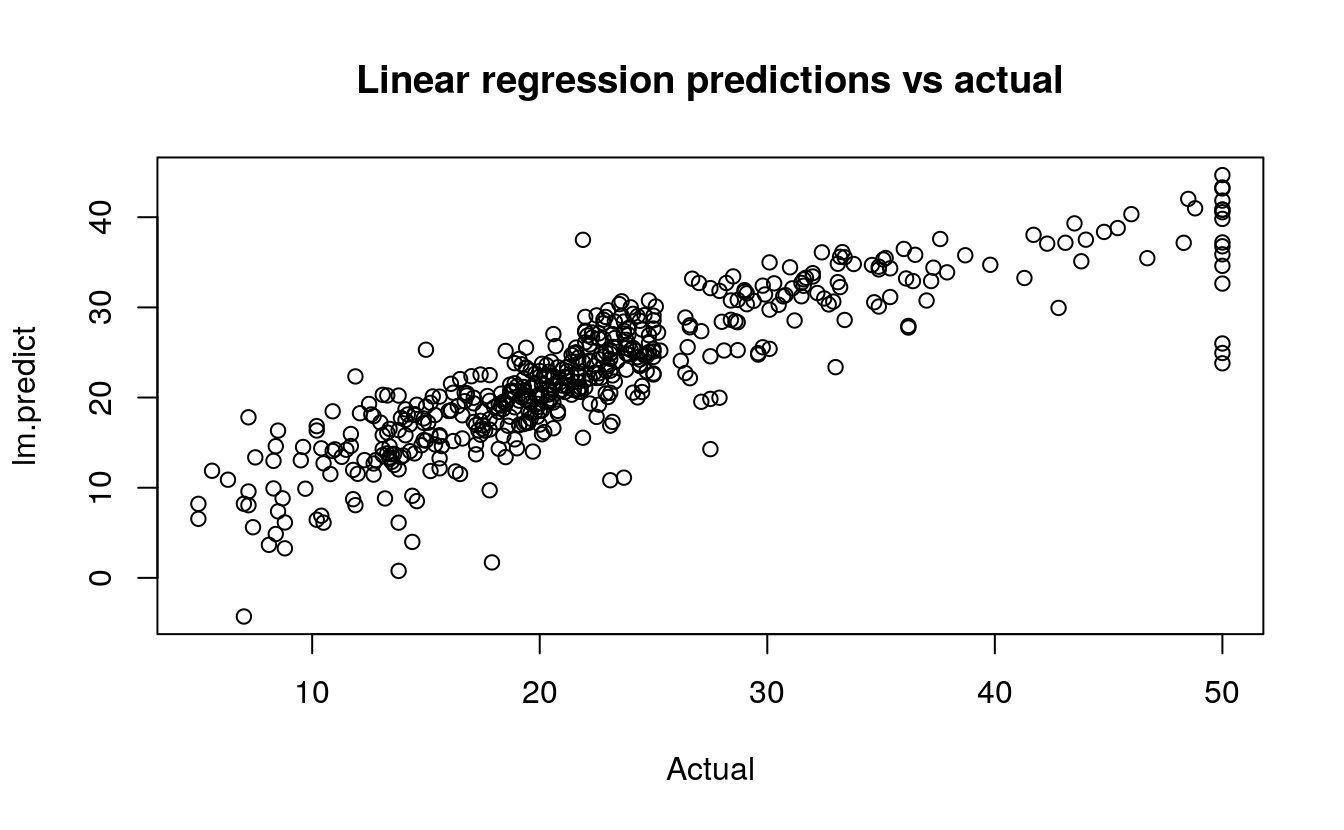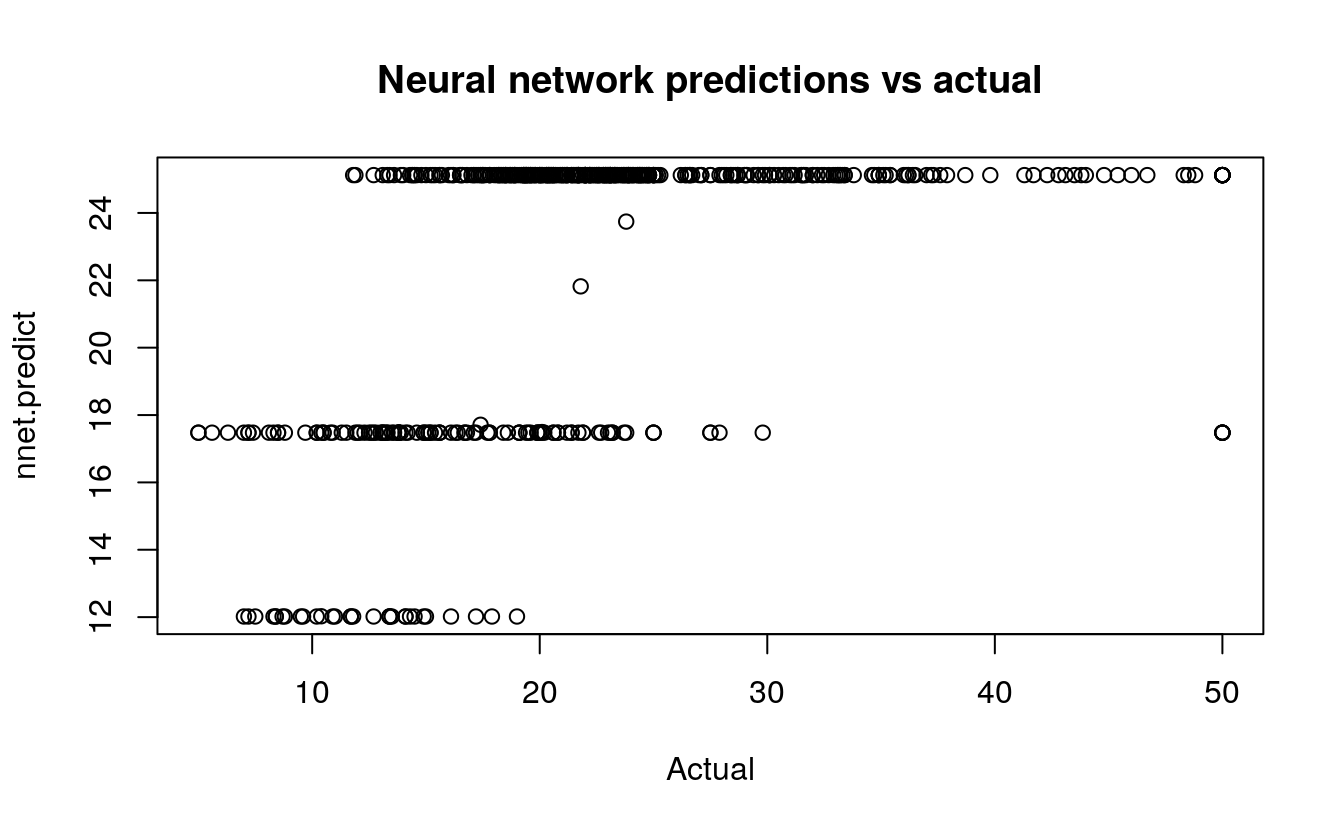# 36 Regression with a neural network

• Dataset: BostonHousing
• Algorithms:
• Neural Network (nnet)
• Linear Regression
###
### prepare data
###
library(mlbench)
data(BostonHousing)

# inspect the range which is 1-50
summary(BostonHousing$medv) #> Min. 1st Qu. Median Mean 3rd Qu. Max. #> 5.0 17.0 21.2 22.5 25.0 50.0 ## ## model linear regression ## lm.fit <- lm(medv ~ ., data=BostonHousing) lm.predict <- predict(lm.fit) # mean squared error: 21.89483 mean((lm.predict - BostonHousing$medv)^2)
#>  21.9

plot(BostonHousing$medv, lm.predict, main="Linear regression predictions vs actual", xlab="Actual")## ## model neural network ## require(nnet) #> Loading required package: nnet # scale inputs: divide by 50 to get 0-1 range nnet.fit <- nnet(medv/50 ~ ., data=BostonHousing, size=2) #> # weights: 31 #> initial value 17.039194 #> iter 10 value 13.754559 #> iter 20 value 13.537235 #> iter 30 value 13.537183 #> iter 40 value 13.530522 #> final value 13.529736 #> converged # multiply 50 to restore original scale nnet.predict <- predict(nnet.fit)*50 # mean squared error: 16.40581 mean((nnet.predict - BostonHousing$medv)^2)
#>  66.8

plot(BostonHousing\$medv, nnet.predict,
main="Neural network predictions vs actual",
xlab="Actual")## 36.1 Neural Network

Now, let’s use the function train() from the package caret to optimize the neural network hyperparameters decay and size, Also, caret performs resampling to give a better estimate of the error. In this case we scale linear regression by the same value, so the error statistics are directly comparable.

 library(mlbench)
data(BostonHousing)

require(caret)

mygrid <- expand.grid(.decay=c(0.5, 0.1), .size=c(4,5,6))
nnetfit <- train(medv/50 ~ ., data=BostonHousing, method="nnet", maxit=1000, tuneGrid=mygrid, trace=F)
print(nnetfit)
#> Neural Network
#>
#> 506 samples
#>  13 predictor
#>
#> No pre-processing
#> Resampling: Bootstrapped (25 reps)
#> Summary of sample sizes: 506, 506, 506, 506, 506, 506, ...
#> Resampling results across tuning parameters:
#>
#>   decay  size  RMSE    Rsquared  MAE
#>   0.1    4     0.0830  0.790     0.0571
#>   0.1    5     0.0814  0.798     0.0559
#>   0.1    6     0.0799  0.806     0.0549
#>   0.5    4     0.0908  0.757     0.0626
#>   0.5    5     0.0897  0.762     0.0622
#>   0.5    6     0.0890  0.766     0.0620
#>
#> RMSE was used to select the optimal model using the smallest value.
#> The final values used for the model were size = 6 and decay = 0.1.
506 samples
13 predictors

No pre-processing
Resampling: Bootstrap (25 reps)

Summary of sample sizes: 506, 506, 506, 506, 506, 506, ...

Resampling results across tuning parameters:

size  decay  RMSE    Rsquared  RMSE SD  Rsquared SD
4     0.1    0.0852  0.785     0.00863  0.0406
4     0.5    0.0923  0.753     0.00891  0.0436
5     0.1    0.0836  0.792     0.00829  0.0396
5     0.5    0.0899  0.765     0.00858  0.0399
6     0.1    0.0835  0.793     0.00804  0.0318
6     0.5    0.0895  0.768     0.00789  0.0344   

## 36.2 Linear Regression

 lmfit <- train(medv/50 ~ ., data=BostonHousing, method="lm")
print(lmfit)
#> Linear Regression
#>
#> 506 samples
#>  13 predictor
#>
#> No pre-processing
#> Resampling: Bootstrapped (25 reps)
#> Summary of sample sizes: 506, 506, 506, 506, 506, 506, ...
#> Resampling results:
#>
#>   RMSE    Rsquared  MAE
#>   0.0988  0.726     0.0692
#>
#> Tuning parameter 'intercept' was held constant at a value of TRUE
506 samples
13 predictors

No pre-processing
Resampling: Bootstrap (25 reps)

Summary of sample sizes: 506, 506, 506, 506, 506, 506, ...

Resampling results

RMSE    Rsquared  RMSE SD  Rsquared SD
0.0994  0.703     0.00741  0.0389    

A tuned neural network has a RMSE of 0.0835 compared to linear regression’s RMSE of 0.0994.